Longest palindromic substring

Not to be confused with Longest palindromic subsequence.

The longest palindromic substring problem is exactly as it sounds: the problem of finding a maximal-length substring of a given string that is also a palindrome. For example, the longest palindromic substring of bananas is anana. The longest palindromic substring is not guaranteed to be unique; for example, in the string abracadabra, there is no palindromic substring with length greater than three, but there are two palindromic substrings with length three, namely, aca and ada. In some applications it may be necessary to return all maximal-length palindromic substrings, in some, only one, and in some, only the maximum length itself. This article discusses algorithms for solving these problems.

Toward a better bound

Thus, the best possible worst-case running time lies between$O(N)$ and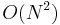$O(N^2)$. We now show that the problem can be solved in$O(N \log N)$ time using standard data structures from the literature, the suffix tree and the suffix array.

Suffix array

The suffix array is a sorted list of the suffixes of a given string, indexed by first character. For example, the suffix array of bananas is [1, 3, 5, 0, 2, 4, 6], corresponding to the suffixes [ananas, anas, as, bananas, nanas, nas, s]. Simple linear-time algorithms now exist for computation of suffix arrays of strings with constant or integer alphabets.

Once the suffix array of a string has been computed, a simple linear-time algorithm will compute the length of the longest common prefix of each pair of adjacent suffixes in the suffix array. The array containing these lengths is known as the lcp array.

If we want to know the longest common prefix of any pair of suffixes, not necessarily lexicographically adjacent, we locate those two suffixes in the suffix array and then find the minimum entry in the lcp array in the range they delimit, hence reducing the problem to a range minimum query. For example, the string teetertotter has suffix array [1,10,4,2,7,11,5,0,9,3,6,8] and lcp array [1,2,1,0,0,1,0,2,3,1,1], because the suffixes starting at positions 1 and 10 have one character in common at the beginning, the suffixes starting at positions 10 and 4 have two characters in common at the beginning, and so on. Suppowe we want to determine the length of the longest common prefix of the suffixes ter (9) and tter (8), which are not adjacent in the suffix array (it is irrelevant that they are adjacent in the string itself). We locate 6 and 8 in the suffix array, and we see from the lcp array that the longest common prefixes of the pairs of suffixes starting at locations 9 and 3, 3 and 6, and 6 and 8 have lengths 3, 1, and 1, respectively. This is a contiguous range of the lcp array. The minimum value in this array, 1, is the answer.

To solve the problem at hand, we will concatenate our string with a unique separator and a reversed copy of itself, so that rearrangement becomes, for example, rearrangement#tnemegnarraer, and then build the suffix array and longest common prefix array for the new string. Then we iterate through all positions in the original string and find the longest palindromic substring centered at each one. To do so, we mirror the current position across the centre of the string, and then take longest common prefixes.

Even case

To determine the longest palindromic substring centered between the two r's (which must be of even length), we consider the suffixes starting at the underlined locations: rearrangement#tnemegnarraer. We see that the longest common prefix of these two suffixes has length 2 (underlined: rearrangement#tnemegnarraer). But because the second half is a reversed copy of the first half, we know the ra in the second half is actually the ar in the first half just before our current position: rearrangement#tnemegnarraer. Thus we have determined that the longest palindromic substring here has length 4. In general we find the character just after our current position in the original string, and the character just before it in the reversed string, and find the longest common prefix using the lcp array and RMQ.

Odd case

In the odd case, we are centered at some character of the original string. In this case, we examine the suffix starting at the character in the original string immediately following it, and the suffix in the reversed string starting at the character immediately preceding it. If we were centered at the m in rearrangement, then, we would be examining the suffixes starting at the underlined characters: rearrangement#tnemegnarraer. We see that the longest common prefix has length 1, and we reflect the e in the reversed half back onto its position in the first half, hence, rearrangement#tnemegnarraer is the longest palindromic substring centered at the m.

Complexity

Since$O(N)$ positions for the centre are considered, and a range minimum query can be executed in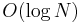$O(\log N)$ time (or constant time with$O(N \log N)$ preprocessing), the time complexity of this solution is$O(N \log N)$.

Suffix tree

The suffix tree is a compressed trie of the suffixes of the string. A detailed discussion of the structure of the suffix tree is given in the article. There exist linear-time algorithms for construction of suffix trees, as well. There are two possible approaches here: we can either concatenate the string with a unique separator and a reversed copy of itself, as with the suffix array, or we can build a generalized suffix tree of the string and its reverse. We proceed as we did with the even and odd cases in the suffix array section, finding longest common prefixes starting at almost-corresponding positions in the original and reverse strings. It is not too hard to see that this is a lowest common ancestor query on the tree. For example, to find the longest palindromic substring of even length centered between the two r's in rearrangement, we could build the suffix tree for rearrangement#tnemegnarraer, locate the leaves corresponding to the suffixes starting at the underlined characters, and find their lowest common ancestor. The depth of this node is the length of the longest common prefix of these two suffixes. Here it is 2, so we know that the substrings underlined match: rearrangement#tnemegnarraer, giving the palindromic substring rearrangement#tnemegnarraer. LCA queries can be executed in constant time after$O(N \log N)$ preprocessing time, giving an$O(N \log N)$ solution.

Existence of a linear-time algorithm

It is possible to execute$O(N)$ LCA queries on a tree with$O(N)$ nodes in$O(N)$ time using a technique due to Gabow and Tarjan. This shows that a linear-time solution exists, which puts to rest the question of the best possible efficiency for a solution to the longest palindromic substring problem.

A simple asymptotically optimal solution

Although we have already established that the$O(N)$ solution using suffix trees and LCA queries is asymptotically optimal, we are driven to seek a better solution for many reasons. The suffix tree based solution:

• requires much code to implement
• has a high constant factor on runtime
• consumes a great deal of memory, and
• is still$O(N \log N)$ with general alphabets.

However, there exists a simpler, shorter, faster, less memory-hungry algorithm which accesses the string only by comparing its characters for equality (and hence has running time independent of alphabet size). This is known as Manacher's algorithm. Again we will compute the longest palindromic substring centered around each possible position (so that returning all longest palindromic substrings is as easy as simply finding the maximum possible length for a palindromic substring). Our algorithm will return a longest palindrome array: an array in which the$i$th element contains the length of the longest palindrome centered around the$i$th position from the left. (Note that the parities of elements in the longest palindrome array alternate.)

The algorithm processes the string from left to right, and fills in the longest palindrome array in the same order, so that when any given position is being considered, the longest palindromes centered around all positions to the left are already known. The algorithm distinguishes two kinds of positions: "interesting" positions and "uninteresting" positions. The algorithm "hops" from each interesting position to the one immediately to the right. It spends amortized constant time on each interesting position. Interesting positions are so named because any maximal-length palindromic substring overall must be centered at one of them. Uninteresting positions' entries in the longest palindrome array can be computed in constant time "in passing" from one interesting position to the next.

We will proceed through a series of observations to derive the algorithm. First, the designation of interesting and uninteresting positions is not unique; in particular, any uninteresting position can be relabelled interesting without affecting correctness or consistency. That being said, we begin by denoting the first position --- the one immediately to the left of the first character --- interesting, and going from there.

Lemma (Second observation): If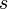$s$ is the longest palindrome centered at the current position, and$t$ is the longest palindromic proper suffix of$s$, then all positions between the current position and the centre of$t$ may safely be denoted uninteresting.

Proof: Consider some position$i$ between the centres of$s$ and$t$. Suppose there exists a palindromic substring,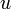$u$, of length greater than or equal to that of$s$, centered at$i$. Certainly the last character of$u$ lies to the right of the last character of$s$, since$u$ is centered further to the right and is longer. So we repeatedly remove one character from each end of$u$ until$u$ and$s$ end at the same position. Since$u$ is now shorter than$s$ (since its centre is to the right but both$s$ and$u$ extend equally far on the right), it is a palindromic proper suffix of$s$. Since the centre of$u$ is to the left of the centre of$t$,$u$ is longer than$t$, a contradiction as$t$ is maximal by hypothesis.

To find the longest palindromic proper suffix, we make yet another observation: the longest palindromic proper suffix and the longest palindromic proper prefix are reflections of each other about the current centre. So we scan backward from the current position. For each position$i$ to the left of the current position, we know the longest palindromic substring$u$ centered there. If the first character of$u$ is to the right of the first character of$s$, then the longest palindromic substring centered at the position obtained by reflecting$u$ about the current position is identical to$s$. (If it were possible to extend it, it would also be possible to extend$s$.) This means we can fill in the corresponding position in the longest palindromic array immediately, and also that there is no palindromic prefix of$s$ centered at$i$ as$u$, and hence no corresponding palindromic suffix at the position obtained by reflecting$i$ across the current centre. Otherwise, on the other hand, we know there is a palindromic (proper) prefix centered at$i$, which means there is a palindromic (proper) suffix centered at the position obtained by reflecting$i$ across the current centre. Furthermore, since we are scanning backward from the current centre, the first such$i$ we encounter is the centre of the longest palindromic proper prefix, so we have also found the longest palindromic proper suffix.

These facts give us enough information to construct an algorithm:

1. (Initialization) Fill in the first entry of the longest palindrome array; it is zero, for this corresponds to the position just before the beginning of the string. Start at the position right on the first character of the string. This is the first interesting position. Also, we need to keep track of the length of the currently longest known palindrome centered at the current position. This starts out as one (the character itself).
2. (Test interesting position) Check whether the palindrome we have so far centered at the current position can be extended (that is, whether there exist identical characters immediately to the left and right of it). If so, extend it. Do this repeatedly until it cannot be extended anymore, either because it is a prefix/suffix of the entire string, or because the next characters on the left and right do not match.
3. We now know the length of the longest palindrome centered at the current location. Fill this in.
4. (Find next interesting position) Let$i$ scan backward through the longest palindrome array and$j$ scan forward, so that$i$ and$j$ are reflections across the current position. Note that the entry for$i$ is always known beforehand.
• If$j$ is after the last position in the string, we have computed all entries in the longest palindrome array, and the algorithm halts. (This always happens eventually.)
• Otherwise, if the longest palindrome centered at$i$ has its first character to the right of the first character of the longest palindrome centered at our current position, then its length$l$ is also the length of the longest palindrome centered at$j$. Fill this in.
• Otherwise, the longest palindrome centered at$j$ is at least as long as the longest palindrome centered at$i$.$j$ is the next interesting position. Go back to step 2, setting the current position to$j$ and the length of the currently longest known palindrome centered there to$l$.

It should now be clear that this algorithm is correct, but it is not necessarily clear that it is efficient.

Theorem: Manacher's algorithm runs in linear time.

Proof:

• The outer loop runs$O(N)$ times.
• Step 1 takes constant time and is executed once.
• Step 2 contains a loop. Each iteration of the loop takes constant time and examines two characters of the string, one on the left of the current palindrome and one on the right. Call the pointer to the next character on the right to examine$r$. After each iteration of the inner loop, either it runs again immediately, moving$r$ one character to the right or step 3 and step 4 follow, which either terminate the algorithm or move the current position to the right, keeping$r$ fixed. (This is because we always move to a suffix in step 4.) So after each iteration either$r$ or the current position is advanced, and thus total number of iterations of the loop in step 2 is no more than the sum of$N$, the number of characters, and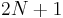$2N+1$, the number of positions. So the total time spent in step 2 is$O(N)$.
• Step 3 takes constant time and is executed once per iteration of the outer loop.
• Step 4 consists of some constant-time parts executed once per iteration of the outer loop, as well as an inner loop. The inner loop takes constant time per iteration can only run$O(N)$ times in total, because each iteration fills in a previously unknown entry in the longest palindrome array, which has$O(N)$ entries.

Overall, each step contributes$O(N)$ to the running time, and so Manacher's algorithm is$O(N)$ overall.